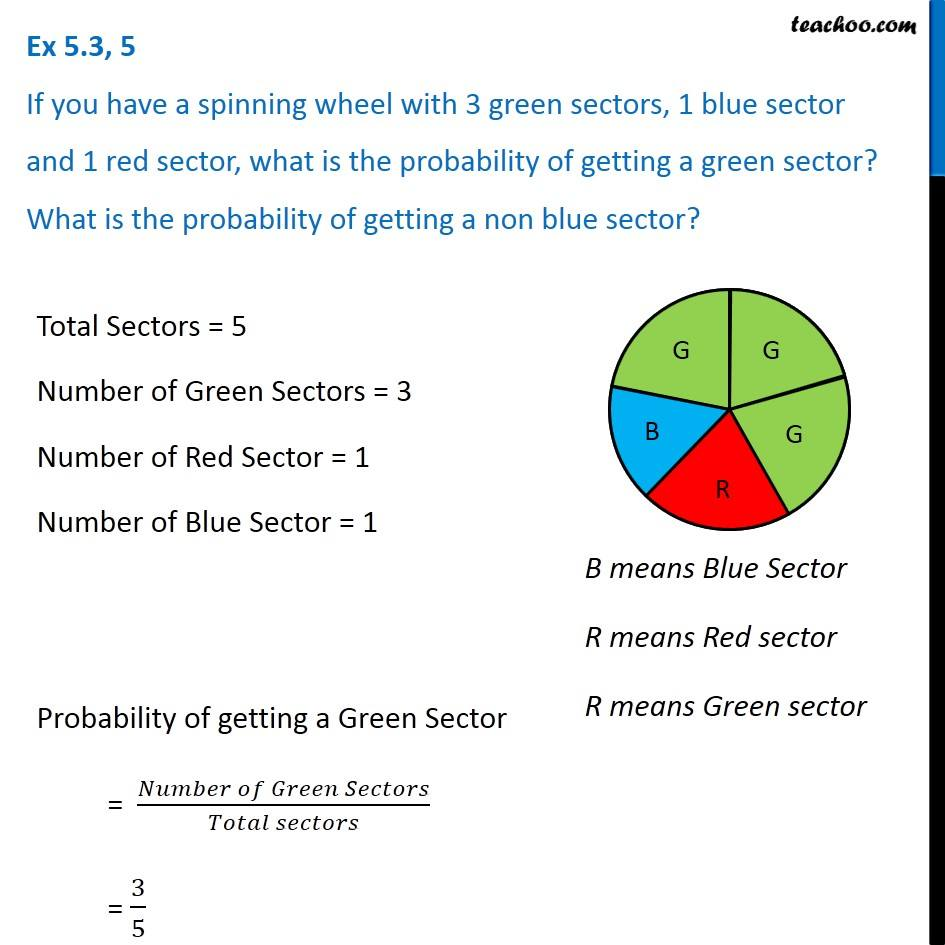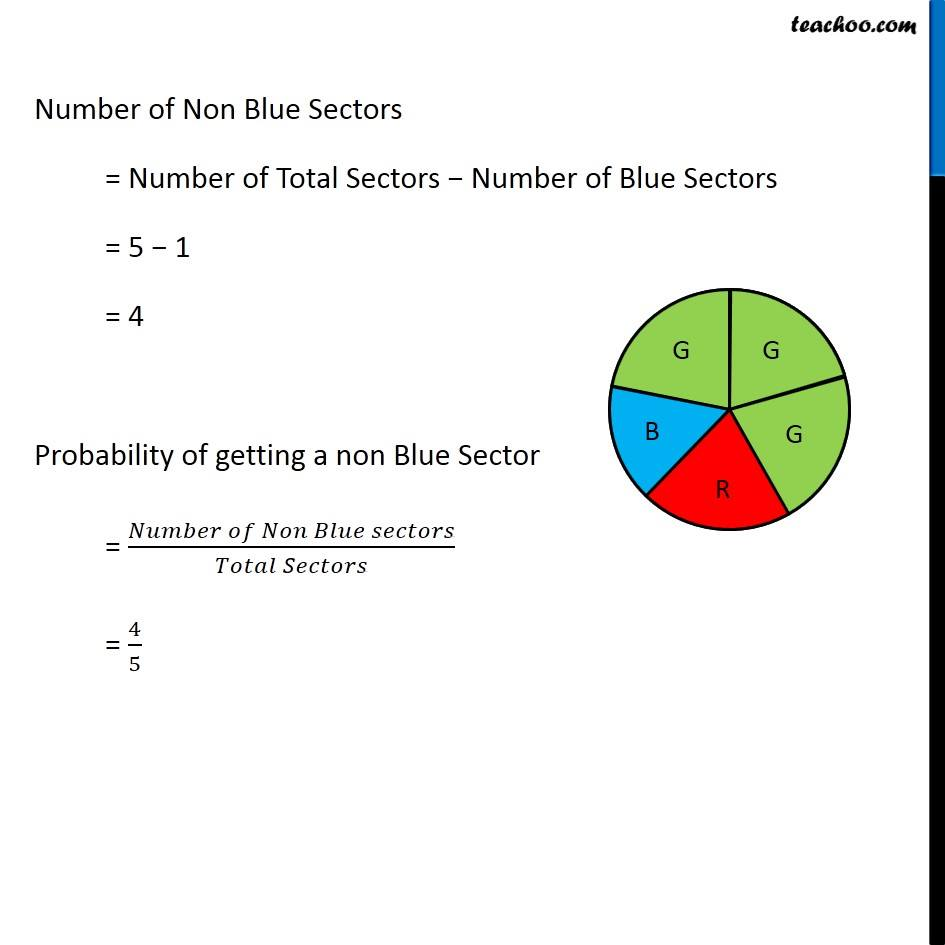Ex 5.3

Chapter 5 Class 8 Data Handling
Serial order wise### Transcript

Ex 5.3, 5 If you have a spinning wheel with 3 green sectors, 1 blue sector and 1 red sector, what is the probability of getting a green sector? What is the probability of getting a non blue sector? Total Sectors = 5 Number of Green Sectors = 3 Number of Red Sector = 1 Number of Blue Sector = 1 Probability of getting a Green Sector = (𝑁𝑢𝑚𝑏𝑒𝑟 𝑜𝑓 𝐺𝑟𝑒𝑒𝑛 𝑆𝑒𝑐𝑡𝑜𝑟𝑠)/(𝑇𝑜𝑡𝑎𝑙 𝑠𝑒𝑐𝑡𝑜𝑟𝑠) = 3/5 Number of Non Blue Sectors = Number of Total Sectors − Number of Blue Sectors = 5 − 1 = 4 Probability of getting a non Blue Sector = (𝑁𝑢𝑚𝑏𝑒𝑟 𝑜𝑓 𝑁𝑜𝑛 𝐵𝑙𝑢𝑒 𝑠𝑒𝑐𝑡𝑜𝑟𝑠)/(𝑇𝑜𝑡𝑎𝑙 𝑆𝑒𝑐𝑡𝑜𝑟𝑠) = 4/5